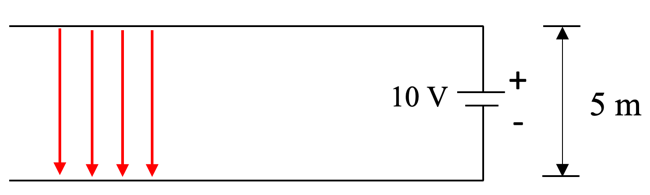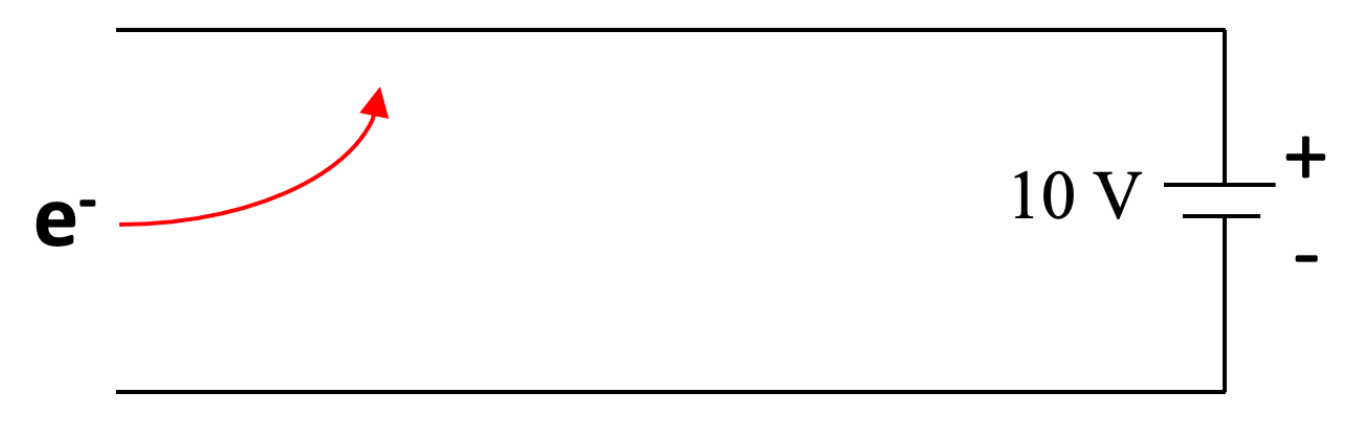# Charged Particle in an Electric Field

This is part of the HSC Physics course under the topic Charged Particles, Conductors and Electric and Magnetic Fields.

### HSC Physics Syllabus

• investigate and quantitatively derive and analyse the interaction between charged particles and uniform electric fields, including: (ACSPH083)
electric field between parallel charged plates E=V/d
acceleration of charged particles by the electric field F_(Net)=ma, F=qE
• model qualitatively and quantitatively the trajectories of charged particles in electric fields and compare them with the trajectories of projectiles in a gravitational field

### Charges in Uniform Electric Fields

This video analyses the interaction between charged particles and uniform electric fields, deriving the relevant syllabus equations. The video also qualitatively and quantitatively compares the trajectory of charged particles in electric fields to that of projectiles in gravitational fields.

### Electric Field Strength

When two parallel charged plates are connected to a potential difference, they produce a uniform electric field with strength:

E=V/d

where,

• E is the electric field strength (Vm-1 or NC-1)
• V is the potential difference or voltage (V)
• d is the perpendicular distance between the two parallel plates (m).
Note: ‘d’ is strictly the distance between two charged plates, it is not dependent on the position of a particle within the electric field.

The direction of the electric field always goes from the positively charged plate to the negatively charged plate, as shown below.Worked Example: In the electric field above, the electric field strength is:

E=10/5=2 Vm-1 downwards

A charged particle experiences a force when in an electric field. Positively charged particles are attracted to the negative plate, and negatively charged particles are attracted to the positive plate. The magnitude of this force is given by the equation:

F_E=qE

where,

• F is the force (N)
• q is the charge of the particle (C)
• E is the electric field strength (Vm-1 or NC-1)

The electric field strength can therefore be also expressed in the form:

E=F/q

Now, since,

E=V/d

then,

F/q=V/d

By Newton’s Second law (F = ma), any charged particle in an electric field experiences acceleration. Hence, their change in displacement increases with time, making their trajectory curved (parabolic) rather than linear.### Qualitative and Quantitative Comparison: Electric and Gravitational Fields

 Feature Motion in Electric Field Motion in Gravitational Field Shape of trajectory Parabolic (for uniform electric fields) Parabolic Is constant force experienced by projectile? Yes, in uniform electric fields. Yes, in uniform gravitational fields. Can be analysed by vector resolution into horizontal and vertical components? Yes Yes Horizontal component of motion Constant Constant What undergoes motion? Charged particles Any type of matter Magnitude of acceleration Typically much larger due to projectiles being of very small mass Typically much smaller, due to projectiles being of larger mass. Magnitude of forces Depends on different parameters than gravitational force.   F = qE (force per unit charge) Depends on different parameters than electric force    F = mg (force per unit mass) Direction of force Depends on nature of charge of projectile: positive particle attracted to negative plate, repelled by positive plate. negative particle attracted to positive plate, repelled by negative plate Always attractive towards centre of mass of source of gravitational attraction.

### Why Gravity is Not Considered for Motion of Electric Charges

Charged particles experience negligible amounts of gravitational force. For example, an electron on the surface of Earth has gravitational force of magnitude:

F_g=(GMm)/d^2

F_g=((6.67xx10^-11)(6.0xx10^24)(9.109xx10^-31))/(6.371xx10^6)^2

F=9.0xx10^-30 N towards the centre of Earth

Compared with typical electric fields, the contribution from electric force is much more significant than gravitational force.

Next section: Work Done in Electric Fields